Next: Evolution of Wave Packets Up: Wave-Particle Duality Previous: Quantum Particles

# Wave Packets

The above discussion suggests that the wavefunction of a massive particle of momentum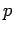and energy, moving in the positive-direction, can be written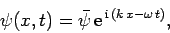(82)

where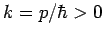and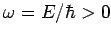. Here,andare linked via the dispersion relation (79). Expression (82) represents a plane wave whose maxima and minima propagate in the positive-direction with the phase velocity. As we have seen, this phase velocity is only half of the classical velocity of a massive particle.

From before, the most reasonable physical interpretation of the wavefunction is thatis proportional to the probability density of finding the particle at positionat time. However, the modulus squared of the wavefunction (82) is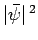, which depends on neithernor. In other words, this wavefunction represents a particle which is equally likely to be found anywhere on the-axis at all times. Hence, the fact that the maxima and minima of the wavefunction propagate at a phase velocity which does not correspond to the classical particle velocity does not have any real physical consequences.

So, how can we write the wavefunction of a particle which is localized in: i.e., a particle which is more likely to be found at some positions on the-axis than at others? It turns out that we can achieve this goal by forming a linear combination of plane waves of different wavenumbers: i.e.,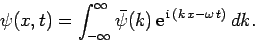(83)

Here,represents the complex amplitude of plane waves of wavenumberin this combination. In writing the above expression, we are relying on the assumption that particle waves are superposable: i.e., it is possible to add two valid wave solutions to form a third valid wave solution. The ultimate justification for this assumption is that particle waves satisfy a differential wave equation which is linear in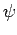. As we shall see, in Sect. 3.15, this is indeed the case. Incidentally, a plane wave which varies asand has a negative(but positive) propagates in the negative-direction at the phase velocity. Hence, the superposition (83) includes both forward and backward propagating waves.

Now, there is a useful mathematical theorem, known as Fourier's theorem, which states that if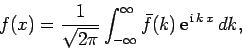(84)

then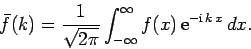(85)

Here,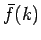is known as the Fourier transform of the function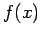. We can use Fourier's theorem to find the-space functionwhich generates any given-space wavefunction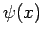at a given time.

For instance, suppose that at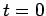the wavefunction of our particle takes the form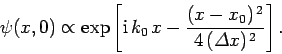(86)

Thus, the initial probability density of the particle is written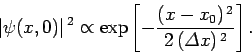(87)

This particular probability distribution is called a Gaussian distribution, and is plotted in Fig. 7. It can be seen that a measurement of the particle's position is most likely to yield the value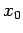, and very unlikely to yield a value which differs fromby more than. Thus, (86) is the wavefunction of a particle which is initially localized around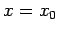in some region whose width is of order. This type of wavefunction is known as a wave packet.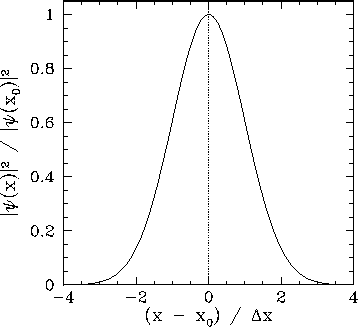Now, according to Eq. (83),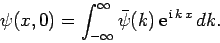(88)

Hence, we can employ Fourier's theorem to invert this expression to give(89)

Making use of Eq. (86), we obtain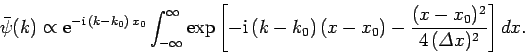(90)

Changing the variable of integration to, this reduces to(91)

where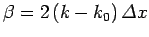. The above equation can be rearranged to give(92)

where. The integral now just reduces to a number, as can easily be seen by making the change of variable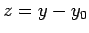. Hence, we obtain(93)

where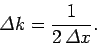(94)

Now, ifis proportional to the probability density of a measurement of the particle's position yielding the valuethen it stands to reason thatis proportional to the probability density of a measurement of the particle's wavenumber yielding the value. (Recall that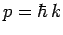, so a measurement of the particle's wavenumber,, is equivalent to a measurement of the particle's momentum,). According to Eq. (93),(95)

Note that this probability distribution is a Gaussian in-space. See Eq. (87) and Fig. 7. Hence, a measurement ofis most likely to yield the value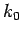, and very unlikely to yield a value which differs fromby more than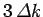. Incidentally, a Gaussian is the only mathematical function in-space which has the same form as its Fourier transform in-space.

We have just seen that a Gaussian probability distribution of characteristic widthin-space [see Eq. (87)] transforms to a Gaussian probability distribution of characteristic width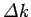in-space [see Eq. (95)], where(96)

This illustrates an important property of wave packets. Namely, if we wish to construct a packet which is very localized in-space (i.e., ifis small) then we need to combine plane waves with a very wide range of different-values (i.e.,will be large). Conversely, if we only combine plane waves whose wavenumbers differ by a small amount (i.e., ifis small) then the resulting wave packet will be very extended in-space (i.e.,will be large).Next: Evolution of Wave Packets Up: Wave-Particle Duality Previous: Quantum Particles
Richard Fitzpatrick 2010-07-20